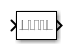# PWM

Generate ideal pulse width modulated signal corresponding to input duty cycle

• Library:

•## Description

Use the PWM block to generate an ideal pulse width modulated signal.

Pulse Width Modulation (PWM) is a technique for encoding an analog signal using square pulses. This encoding is achieved by controlling the fraction of one period of the square wave that is set to high. This fraction is the duty cycle of the signal. The relationship between the modulated signal and the input duty cycle can be simply described as:

`$\overline{y}=D{y}_{max}+\text{​}\left(1-D\right){y}_{min}$`

where ymax and ymin are the upper and lower bounds of the duty cycle, respectively. For the PWM block, the duty cycle is constrained to [0,1]. Thus the idealized PWM signal is proportional to the duty cycle D.

## Ports

### Input

expand all

Desired duty cycle of the pulse P, specified as scalar within the range [0,1].

Data Types: `double`

### Output

expand all

PWM signal corresponding to the input duty cycle.

Data Types: `double`

## Parameters

expand all

Time between rising edges of consecutive pulses of the output signal. A smaller value represents a higher frequency pulse.

Select whether the block should operate in continuous or discrete sampling modes.

By default, the block uses `continuous` sampling mode as it improves simulation performance with variable step solvers.

Select `discrete` sampling mode if you need to:

• use a fixed-step solver

• generate code

• sample the block output

Specify the rate at which the block samples input duty cycle signal. This sampling rate becomes the resolution of the output pulse signal.

#### Dependencies

This parameter requires that Sampling mode is set to `discrete`

Enable this parameter to break algebraic loops containing the PWM block.

Note

Enabling this parameter casues a signal value of 0 or below for the Duty Cycle input to throw an error.

Data Types: `Boolean`

## Block Characteristics

 Data Types `double` Direct Feedthrough `no` Multidimensional Signals `no` Variable-Size Signals `no` Zero-Crossing Detection `no`

expand all